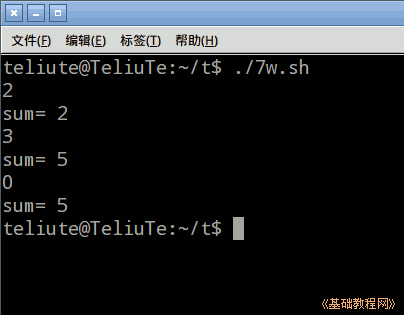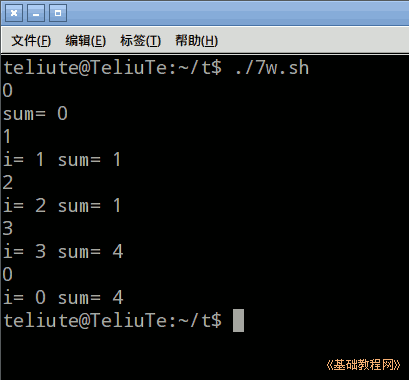Bash Shell 脚本编程基础

while 循环一般用在不能确定循环次数的情况，根据条件来循环；

1、while 循环

1）启动文本编辑器gedit，输入第一行 #!/bin/bash ，保存文件为 7w.sh，并设置可执行权限；

#!/bin/bash

2）语句格式为 while [条件]; do 命令; done ，输入下面的语句；

#!/bin/bash
i=1
while (( \$i!=0 ))
do
((sum=sum+i))
echo "sum= \$sum"
done

条件也可以用方框[ \$i -ne 0 ] ，程序运行后，输入一个数按回车，如果输入的是0，就会结束程序；3）循环中的 break 语句用于退出循环，continue 语句用于结束本次循环，直接进行下一次循环，输入下面的语句；

i=1
while [ \$i -ne 0 ] #(( \$i!=0 ))
do
if((i%2==0))
then
echo "i= \$i sum= \$sum"
continue
fi
((sum=sum+i))
echo "i= \$i sum= \$sum"
done

%是取余，偶数只显示，奇数加到sum里；4）下载本课范例

本节学习了while 循环的基础知识，如果你成功地完成了练习，请继续学习下一课内容；